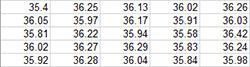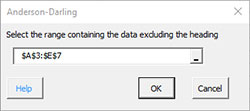# Normal Probability Plot HelpThis test helps determine if your data are normally distributed. Data that are normally distributed will fall close to a straight line on the normal probability plot. The Anderson-Darling test calculates a statistic that helps detect most departures from normality.

If the p value (probability) for the Anderson-Darling statistic is less than 0.05, there is statistical evidence that the data are not normality distributed. If the p value is greater than 0.20, the conclusion is that the data are normally distributed. More data might be needed for values of p between 0.05 and 0.20. An example is given below.

A hardness tester’s calibration is checked by testing a standard block once a day. The measurement error should be normally distributed. Data for the last 25 days is given below. That data can be downloaded at this link. We want to determine if the results are normally distributed.

1. Enter the data into an Excel worksheet. It can be in columns, rows or over several columns and rows.2. Select the data.

3. Select the “Normal Probably Plot from the “Distributions” panel in the SPC for Excel ribbon.### Normal Probability Plot Output

The program will generate the normal probability plot with the Anderson-Darling statistic as shown below. The points generally lie along the straight line on the normal probability plot. The Anderson-Darling statistic is printed along with the p value, which in this case is 0.2195. Since it is greater than 0.20, you conclude that the data come from a normal distribution.Notes:

• The Anderson-Darling statistic is the value of AD*, the corrected value for sample size.